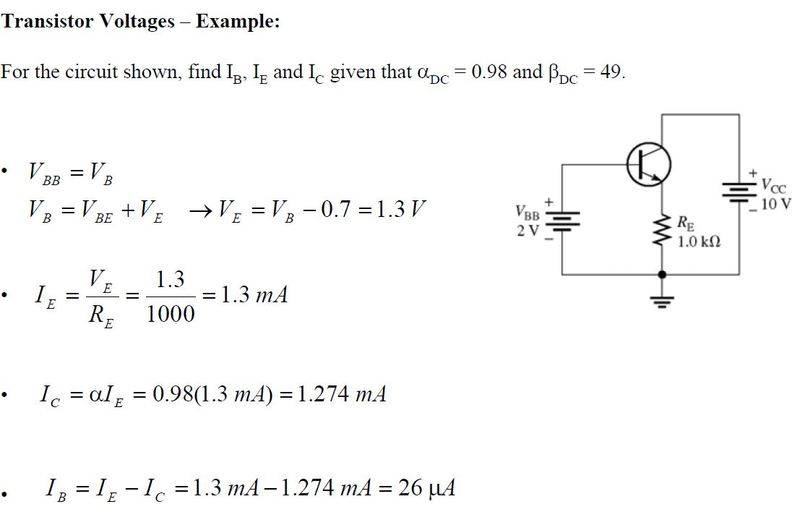BJT voltage on the emitter question

i got this example from our lecture notes, there is one thing i dont understand,

When calculating Ve, he takes Vb and minus the Voltage drop across transistor, so 2V - 0.7V and gets 1.3 V for Ve,

my confusion is why did he ignore Vc? should it not be (Vc + Vb)-0.7V ,so (10V+2V)-0.7V=11.3V on Ve ?

thanksWhat you have done is fine. There is another voltage Vce which you have not been asked to calculate.
This will be Vcc - Ve = 10 - 1.3 = 8.7V

vk6kro
Vcc isn't in series with Vbb in this diagram.

Try to think of it as two loops with the current in the left loop controlling the current in the right loop. The actual value of Vcc doesn't matter much as long as it can supply the necessary current for the right loop.

When the circuit is turned on, base current starts to flow from Vbb. This turns the transistor on and collector current starts to flow in the right loop.

This (and a small contribution from the base current) produces a voltage across the emitter resistor which rises until the sum of this voltage and the base-emitter voltage equals Vbb.

At this point, the base current becomes steady and a predictable collector current is flowing.

Vcc isn't in series with Vbb in this diagram.

Try to think of it as two loops with the current in the left loop controlling the current in the right loop. The actual value of Vcc doesn't matter much as long as it can supply the necessary current for the right loop.

When the circuit is turned on, base current starts to flow from Vbb. This turns the transistor on and collector current starts to flow in the right loop.

This (and a small contribution from the base current) produces a voltage across the emitter resistor which rises until the sum of this voltage and the base-emitter voltage equals Vbb.

At this point, the base current becomes steady and a predictable collector current is flowing.
aha! that makes alot of sense, thanks alot!